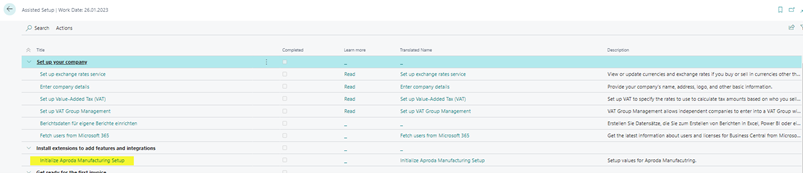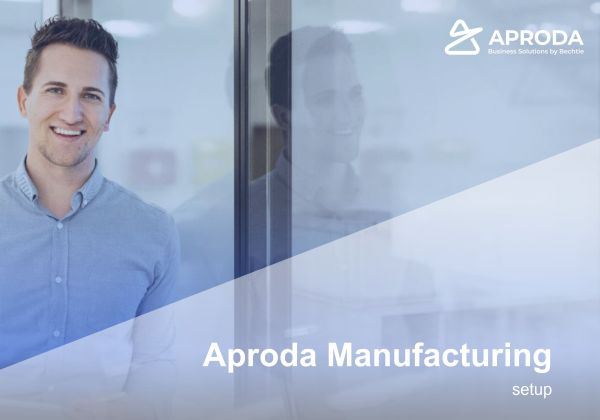## Aproda Manufacturing

Aproda Manufacturing can be used to map the following processes:

• Create pre-calculation for production order (Simulate scenarios without editing master data, using field to modify calculation lines)
• Create post-calculation for one or more production orders
• Calculation summary sheme (direct material cost, indirect material cost, production cost per piece, production overheads, scrap cost, external production cost, selling & administrative overheads, manufacturing cost, cost of goods)
• Detailled breakdown of cost: Items and routing-lines
• Summary cockpit-view for pre- and post-calculations
Assisted Setup

#### 1. Starting the Assisted Setup

For the first time use Aproda Manufacturing, you can initialize the setup using the assisted setup.

1. Step: Open the assisted setup

2. Step: Select initialize Aproda Manufacturing SetupAproda Manufacturing Setup

See the setup of the Aproda Manufacturing App below

Calculation

1. Calculation header (fields independent from type Pre- and PostCalculation)

Calculations are used to calculate cost for production orders, as PreCalculation for estimated cost or PostCalculation for real cost. Setup Guide >>

2. Pre Calculation

Find the setup guide for the Pre Calculation here >>

3. Post Calculation

Find the setup guide for the Post Calculation here >>

Calculation cockpit

The Calculation Cockpit gives summary view for pre- and post-calculations.